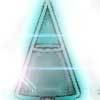#### You may also like### Matter of Scale

Can you prove Pythagoras' Theorem using enlargements and scale factors?### Conical Bottle

A right circular cone is filled with liquid to a depth of half its vertical height. The cone is inverted. How high up the vertical height of the cone will the liquid rise?### Squirty

Using a ruler, pencil and compasses only, it is possible to construct a square inside any triangle so that all four vertices touch the sides of the triangle.

# Similar Rectangles

##### Age 14 to 16Challenge Level

The smaller of two similar rectangles has height $2$ units; the larger rectangle has length $6$ units.

If one rectangle has twice the area of the other, find the length of the smaller rectangle.

This problem is taken from Tony Gardiner's 'Extension Mathematics Gamma' book.